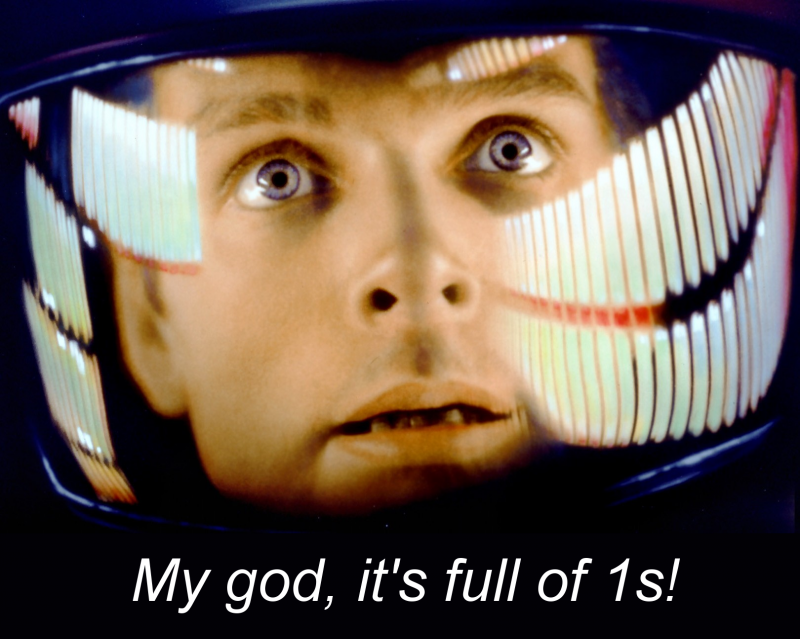# You're reading: Posts Tagged: number theory

### 33 can be written as the sum of three cubes

It was an open question whether 33 could be written as the sum of three cubes. Thanks to Andrew R. Booker, it now isn’t.

\begin{array}{c} (8866128975287528)^3 \\ + \\(-8778405442862239)^3 \\ + \\(-2736111468807040)^3 \\ = \\ 33\end{array}

### Abel Prize 2018

The Abel Prize for 2018 has been awarded to Robert Langlands, for his work on representation theory and number theory. The Abel Prize website has a page with more information, including a lay explanation of Langlands’ work by Alex Bellos.

### $2^{77,232,917}-1$ is the new $2^{74,207,281}-1$We now know 50 Mersenne primes! The latest indivisible mammoth, $2^{77,232,917}-1$, was discovered by Great Internet Mersenne Prime Search user Jonathan Pace on the 26th of December 2017. As well as being the biggest Mersenne prime ever known, it’s also the biggest prime of any sort discovered to date.

GIMPS works by distributing the job of checking candidate numbers for primality to computers running the software around the world. It took over six days of computing to prove that this number is prime, which has since been verified on four other systems.

Pace, a 51-year old Electrical Engineer from Tennessee, has been running the GIMPS software to look for primes for over 14 years, and has been rewarded with a \$3,000 prize. When a prime with over 100 million digits is found, the discoverer will earn a \$50,000 prize. That probably won’t be for quite a while: this new prime has $23{,}249{,}425$ decimal digits, just under a million more than the previous biggest prime, discovered in 2016.

If you’re really interested, the entire decimal representation of the number can be found in a 10MB ZIP file hosted at mersenne.org. Spoiler: it begins with a 4.

More information: press release at mersenne.org, home of the Great Internet Mersenne Prime Search.

via Haggis the Sheep on Twitter

### 13532385396179 doesn’t climb to a prime

Someone called James Davis has found a counterexample to John H. Conway’s “Climb to a Prime” conjecture, for which Conway was offering \$1,000 for a solution. The conjecture goes like this, as stated in Conway’s list of \$1,000 problems:

Let $n$ be a positive integer. Write the prime factorization in the usual way, e.g. $60 = 2^2 \cdot 3 \cdot 5$, in which the primes are written in increasing order, and exponents of $1$ are omitted. Then bring exponents down to the line and omit all multiplication signs, obtaining a number $f(n)$. Now repeat.

So, for example, $f(60) = f(2^2 \cdot 3 \cdot 5) = 2235$. Next, because $2235 = 3 \cdot 5 \cdot 149$, it maps, under $f$, to $35149$, and since $35149$ is prime, we stop there forever.

The conjecture, in which I seem to be the only believer, is that every number eventually climbs to a prime. The number 20 has not been verified to do so. Observe that $20 \to 225 \to 3252 \to 223271 \to \ldots$, eventually getting to more than one hundred digits without reaching a prime!

Well, James, who says he is “not a mathematician by any stretch”, had a hunch that a counterexample would be of the form $n = x \cdot p = f(x) \cdot 10^y+p$, where $p$ is the largest prime factor of $n$, which in turn motivates looking for $x$ of the form $x=m \cdot 10^y + 1$, and $m=1407$, $y=5$, $p=96179$ “fell out immediately”. It’s not at all obvious to me where that hunch came from, or why it worked.

The number James found was $13\,532\,385\,396\,179 = 13 \cdot 53^2 \cdot 3853 \cdot 96179$, which maps onto itself under Conway’s function $f$ – it’s a fixed point of the function. So, $f$ will never map this composite number onto a prime, disproving the conjecture. Finding such a simple counterexample against such stratospherically poor odds is like deciding to look for Lord Lucan and bumping into him on your doorstep as you leave the house.

A lovely bit of speculative maths spelunking!

via Hans Havermann, whom James originally contacted with his discovery.

### Small gaps between large gaps between primes results

The big news last year was the quest to find a lower bound for the gap between pairs of large primes, started by Yitang Zhang and carried on chiefly by Terry Tao and the fresh-faced James Maynard.

Now that progress on the twin prime conjecture has slowed down, they’ve both turned their attentions toward the opposite question: what’s the biggest gap between subsequent small primes?

### From the Mailbag: Dual Inversal Numbers

Katie, one of our editors, has been contacted by Brendan with a question about some maths he’s been investigating. Read on to find out what he’s discovered, and read Katie’s response.Dear The Aperiodical,

I’ve noticed an interesting property of numbers, and I wondered if you could tell me if this is something which is already known to mathematicians? I’ve been calling them Dual Inversal Numbers, but I’d love to know if they have an existing name, and if there’s anything else you can tell me about them.

### Cushing your luck: properties of randomly chosen numbers

Long-time Aperiodical muse David Cushing has made a bet with us that he can give us an interesting post every Friday for the next ten weeks. Every week that he sends a post, we buy him a bar of chocolate. Every week that he doesn’t send us a post, he buys us a bar of chocolate. For his first trick, David is going to do some unnatural things with the natural numbers.

The greatest common divisor (gcd) of two or more integers is the greatest integer that evenly divides those integers. For example, the gcd of $8$ and $12$ is $4$ (usually written as $\gcd(8,12)=4$). Two integers are called coprime (or “relatively prime”) if their gcd is equal to $1$.

A reasonable question to ask is,

Given two randomly chosen integers $a$ and $b$, what is the probability that $\gcd(a,b)=1$?